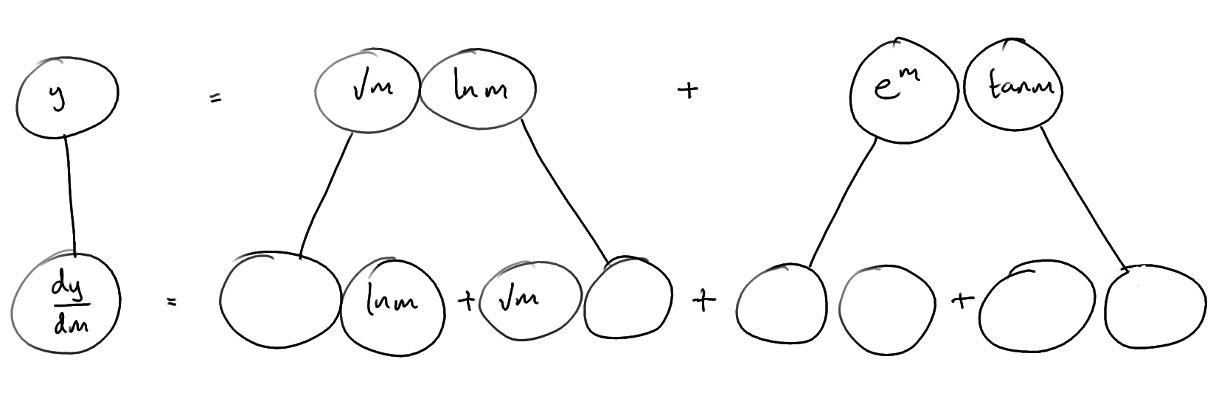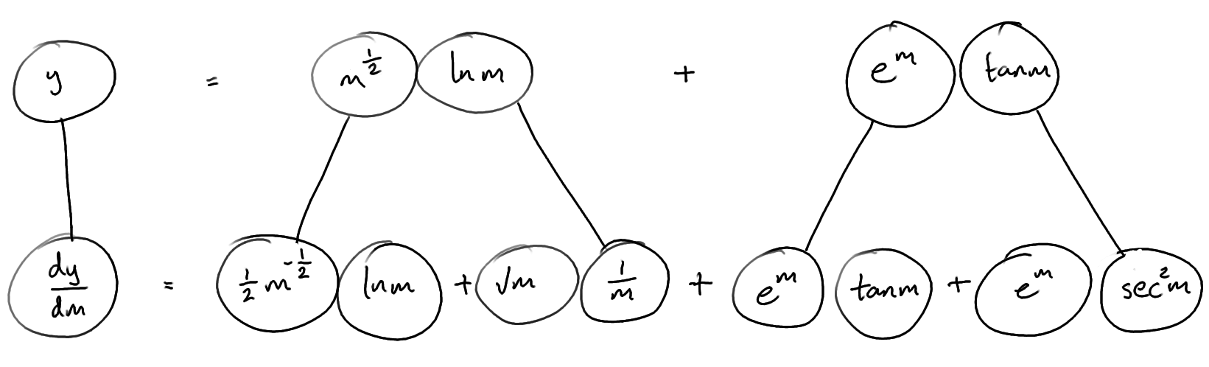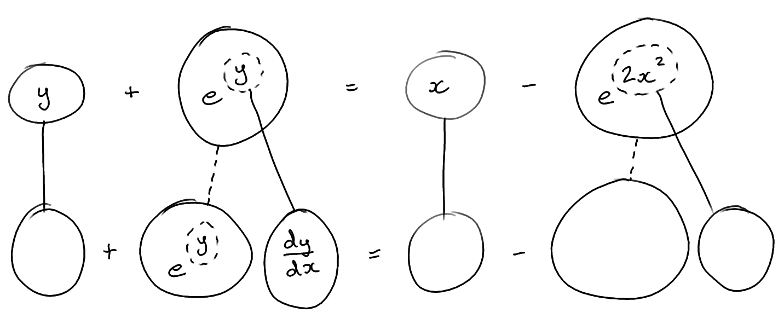# Thread: Differentation question

1. ## Differentation question

Hi guys. Struggling with some revision questions.
So if you could step out how you solved the questions, that'd be amazing.

firstly;

y(m) = (sqrt m) ln m + e^m tan m
(brackets just to show that the square root symbols only covers the m)

and

y + e^ t = m - e^(2m^2)

please help!

2. ## Re: Differentation question

Just in case a picture helps...... where (key in spoiler) ...

Spoiler:... is the product rule. Straight continuous lines differentiate downwards (integrate up) with respect to m. And,

Spoiler:Second... is t a function of m? Full question please.

_________________________________________

Don't integrate - balloontegrate!

Balloon Calculus; standard integrals, derivatives and methods

Balloon Calculus Drawing with LaTeX and Asymptote!

3. ## Re: Differentation question

sorry typed the question in wrong. the question is y + e^y = x-e^2x^2

so from your answer i should be getting $\displaystyle dy/dm = 1/2 m^-(1/2) ln m + sqrt(m) 1/m + e^m tan m + e^m sec^2 m$ ?

4. ## Re: Differentation question

so from your answer i should be getting $\displaystyle dy/dm = 1/2 m^-(1/2) ln m + sqrt(m) 1/m + e^m tan m + e^m sec^2 m$ ?
Yes - see second 'spoiler' above.Originally Posted by Hooperoosorry typed the question in wrong. the question is y + e^y = x-e^2x^2
Right, so it's impicit differentiation using the chain rule...... where (key in spoiler) ...

Spoiler:... is the chain rule. Straight continuous lines differentiate downwards (integrate up) with respect to the main variable (in this case x), and the straight dashed line similarly but with respect to the dashed balloon expression (the inner function of the composite which is subject to the chain rule).

Spoiler:Now you may want to 'solve' for dy/dx, i.e. factorise the left hand side (of the bottom row) and then divide both sides by (1 + ey), to make dy/dx the 'subject'.

_________________________________________

Don't integrate - balloontegrate!

Balloon Calculus; standard integrals, derivatives and methods

Balloon Calculus Drawing with LaTeX and Asymptote!

5. ## Re: Differentation question

so i should be going from

$\displaystyle {dy/dm} = 1/2 m^-{1/2} ln m + sqrt(m) {1/m} + e^{m} tan m + e^{m} sec^{2} m$?

to

$\displaystyle {dy/dx} + e^{y} {dy/dx} = 1- e^{2x^{2}} 4x$

?

6. ## Re: Differentation question

Sure... if, for some reason or other, you want to be going from

the answer to the first problem

to

the answer to the second

?!

7. ## Re: Differentation question

Im sorry sirthis just confused me greatly and i didnt know what i was doing.

ill try harder next time### questions of differentation

Click on a term to search for related topics.

#### Search Tags

differentation, question